5.2 Graphical Representation of Data:

Bar Chart

5.2 Example 1: You or your friend as a cricketer must be scoring runs in your school matches. In one of such match assume you/your friend had scored a century by scoring runs all around the wicket. The scoring must have been across 6 major areas (Midwicket, Cover, Longon, Longoff, Fineleg  & Thirdman) of the wicket. The scoring pattern for 100 runs is given in the table given below:

Workings: Let us draw a table with scoring area and runs scored as shown below:

 Scoring Area Runs Midwicket 28 Cover 20 Longon 25 Longoff 9 Fineleg 11 Thirdman 7 Total 100

This data can be pictorially represented as shown in the adjoining figure (Bar chart).

The vertical line has markings in steps of 5 runs (from 0 to 30).

The names of the area are marked on the horizontal line and each area is represented by a bar.

The height(length) of the bar is proportional to runs scored.

Width of the bars is same.

The space between bars is same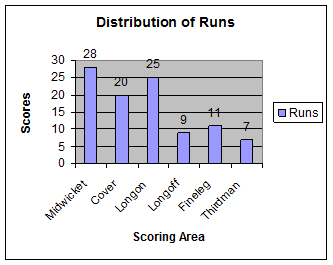Pie Chart

 Let us represent the above data in a different format as shown in the adjoining figure called Pie Chart.   Here the areas are represented by different colors, starting with Midwicket area, the area in which highest runs were scored (28%). In this type of chart, runs are represented by the size of the sector converted to percentage.   Higher the percentage, larger will be the size of the sector (converted to percentages).   If you compare the above two types of pictorial representations for the same data (Bar chart and Pie chart), don’t you think that Pie Chart is more appropriate as compared to Bar chart in this example.             Why?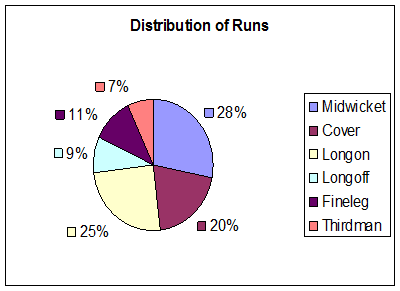The reason is Pie chart gives us a complete picture of scoring pattern and sum of percentages of all sectors = 100%. Bar chart is suitable when the data values expressed as %, do not add up to 100% (like in the case of data for several time periods, countries etc)

5.2 Example 2: Let us consider the following example of results of a Board examination given in pass percentage for few years as given below:

 Year Pass% 2001 44.2 2002 48.0 2003 46.5 2004 47.0 2005 44.5

In the adjoining figure, we have marked pass % from 42 and not from 0.

(If done from 0, the graph would have become too big)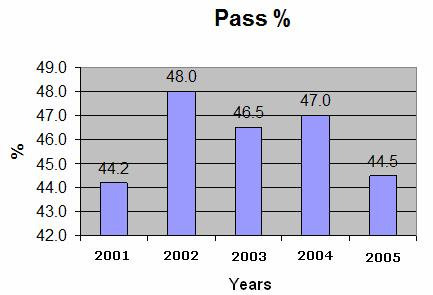Construction of Bar and Pie charts

5.2. Example 3:  Draw a suitable chart to represent the following data about heartbeat of a human being.

 Age in years            à 5 10 12 15 Above 18 Heart beats/ Minute à 100 90 85 80 72

Solution:

 We notice that heartbeats can not be expressed as % and hence Pie chart can not be drawn for this set of data. We have to represent this data as Bar chart. Step1. On a sheet of paper or graph sheet, draw a horizontal line OX, Step2.  Draw a vertical line OY perpendicular to OX at O OX will be the line which will represent Ages and OY will be the line which will represent heartbeats. Step 3. Since heartbeats are between72 and 100, we use the scale 1cm = 10 heartbeats. So we have 100 hearbeats =  100*1cm/10 = 10cm 92   heartbeats =   92*1cm/10 = 9.2cm 85   heartbeats = 85*1cm/10   = 8.5cm 80   heartbeats = 80*1cm/10  =  8cm 72   heartbeats = 72*1cm/10  = 7.2cm These will be the heights of bars. Step 4. On line OX, mark ‘5’ at approximately 1.5cm  away from O (this will represent the age group 5). Step 5. Construct a bar of height 10cm (=100 heartbeats) and width of 1cm above ‘5’. This bar will represent the age group 5. Step 6. Mark ‘10’ at approximately 1.5cm away from the above bar (this will represent the age group 10). Step 7. Construct a bar of height 9cm(=90 heartbeats) and width of 1cm above ‘10’. This bar will represent the age group 10. Step 8. Repeat steps 6 and 7 for the remaining age groups of 12, 15 and ‘>18’ with heights of bar equal to 8.5cm, 8cm and 7.2cm respectively. Width of the bar will be  same(1cm)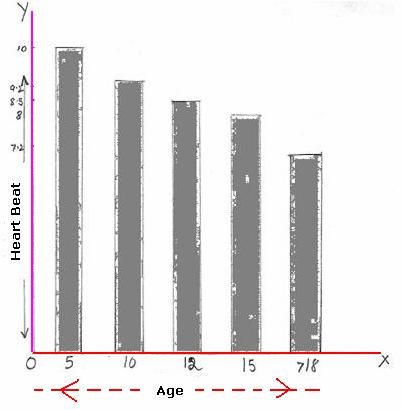5.2 Example 4: Draw a chart for viewer ship (viewing pattern) of different channels, among 250 students, which is given below.

 No Channel Students 1 National Geographic 100 2 Discovery Channel 50 3 Sports Channel 75 4 Others 25

Solution:

Since students belonging to each category can be expressed as % of the  total (250), we need to draw a Pie Chart.

Step 1 : Calculate the size of sector proportionate to the number of students for each of the above 4 categories.

Note : Angle at center of circle is 3600.

 Channel Students Angle of the sector National Geographic 100 (360/250)*100 = 1440 Discovery Channel 50 (360/250)*50   =  720 Sports Channel 75 (360/250)*75   = 1080 Others 25 (360/250)*25   =   360 Total --à 250 3600

We multiplied each of the student count by 360 and divided them by total number of students (250) to get the proportionate size of the sector.

Step2. Draw a circle of suitable radius

Step3. Mark the above angles at the center of the circle and draw sectors correspondingly.

Step4. Shade them suitably so as to distinguish each of the sectors and write names of the channel in the appropriate sector.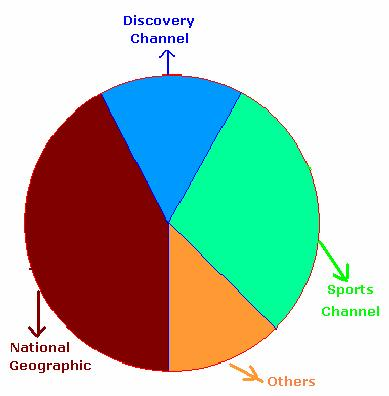5.2 Example 5: The four sections in 10th standard of a school have below mentioned class strength

 Section Girls Boys Total A 24 36 60 B 27 30 57 C 23 40 63 D 19 35 54

Represent the above data in a suitable format.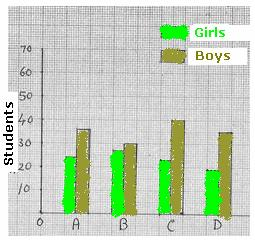We could draw two bar charts for the above data (One for boys, one for girls separately)   In such a case since charts are different, it would not be possible to read and compare data easily.   If we represent data for girls and boys side by side as in figure on the left hand side it becomes easy for analysis. Such a bar chart is called ‘Joint Bar Diagram (chart)’   As an alternative, what if we represent data in the same bar one above the other, like in the figure on the right hand side? Such a bar chart is called ‘Sub-divided bar chart’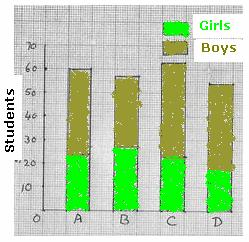5.2 Summary of learning

 No Points to remember 1 Bar chart is a method of representation of data in bars of equal width. However length of the bar is proportional to its figure (number). 2 Pie chart is a method of representation of data in terms of sectors of a circle. The size of sector is proportional to its figure (number).